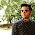Friday, 14 February 2014

Be my mathematical Valentine.

Looking for an unusual gift to give your love this Valentine's day? Is the significant figure in your life a mathematician? Realise that you haven't bought anything and quickly need to knock something up? Then look no further as what could be more romantic than mathematics?

The simplest thing you could do is text the one you love with a simple I <3 U'. However, if your partner really is a mathematician, then I would suggest sending I/3 < U' instead and let them work out the message.

For those who are willing to go that extra mile (and have Matlab) here are a few suggestions of how to produce a Valentine token with a uniquely numerical twist.

1) The easiest method way to produce a heart is to use parametrized functions as seen in Figure 1. In fact the code to produce the heart in Figure 1 is so simple that I decided to use Matlab's annotation facilities to add an arrow to the picture. The whole code to produce this image can be found below.Figure 1. Produce a number of values of t spanning $-\pi$ to $\pi$. Calculate the appropriate values of x and y and, finally, plot them.

2) A slightly different heart shape can be produced using an implicitly defined set of points given by the equation shown in Figure 2. This equation is slightly harder to plot because given a value of x you have to solve a cubic equation to work out the corresponding value of y. Thankfully, we're able to let Matlab bare the brute force work and simply plot the delightful result.Figure 2. Unlike the previous equation were we were able to calculate x and y explicitly, this equation has no such nice closed form solution, or parametrization.
3) For those of you with a love that cannot be contained within two dimensions there is also a three-dimensional heart shape shown in Figure 3. Once again, this is slightly more difficult than the previous example. Not only do we have to contend with an implicit equation, but it is in three variables, instead of two! In fact I was unable to plot the equation easily using the basic functionality of Matlab, so I resorted to using one of the files from the file exchange, Ezimplot3.Figure 3. A fully rendered three-dimensional heart produced using Ezimplot3.
4) Finally, the last one has a special place in my heart for a number of reasons. Each year I get my wife n roses for the n years that we have been together. Often I try to vary the type I get. For example, one year I got roses made of wood, another year I got roses made of feathers. Last year I decided to make her a rose using my coding abilities. This is shown in Figure 4.Figure 4. A rose by any other name would smell like a Turing pattern.
Regular readers will no doubt know that Alan Turing's theory of Morphogenesis is my favourite piece of mathematics. So, I used an image of a rose to form the initial condition of a Turing pattern and you can see the whole evolution of the pattern in Figure 4.

As I say, this animation is very special to me, so unlike the other images, I won't be giving the code out for it. However, for the interested party, the coding behind it is not too difficult. All you need to do it to load a grey scale image into Matlab and use the image values as a two-dimensional network on which you run the reaction-diffusion equations.
________________________________________________________________
________________________________________
CODES
CODE 1
%% Clear variables
clear
close
clc

%% Set up figure size
screen_size = get(0,'screensize');
figure_position =[0 0 500 screen_size(4)/1.2];
h=figure('outerposition',figure_position);

%% Variables
fs=50; %Fontsize
t=linspace(-pi,pi,1000);
x=16*sin(t).^3;
y=13*cos(t)-5*cos(2*t)-2*cos(3*t)-cos(4*t);

%% Plot
area(x,y,'facecolor','r','edgecolor','none')

%% Add in the text
text(0,15,'I','fontsize',fs,'HorizontalAlignment','center','color','b')
text(0,0,{'x=16sin(t)^3';'y=13cos(t)-5cos(2t)-2cos(3t)-cos(4t)'},'fontsize',12,'HorizontalAlignment','center','color',[1,1,1])
text(0,-20,'You','fontsize',fs,'HorizontalAlignment','center','color','b')
text(20,-24,'By Thomas E. Woolley','fontsize',fs/7,'HorizontalAlignment','right','color','k')

%% Add in the arrow
annotation('line',[.3 .4],[.4 .4-0.6667*(.3-.4)],'linewidth',5,'color','k')

%% Tidy up the plot
axis equal
axis([-20 20 -25 20])
set(gcf,'PaperPositionMode','auto')

%% Save
print(gcf, '-r300',['./1D.png'], '-dpng');
________________________________________________________________
CODE 2
%% Clear variables
clear
close
clc

%% Set up figure size
screen_size = get(0,'screensize');
figure_position =[0 0 500 screen_size(4)/1.2];
h=figure('outerposition',figure_position);

%% Variables
fs=30; %Fontsize
[x,y] = meshgrid(-3:.01:3);
f = (x.^2+y.^2-1).^3-x.^2.*y.^3;

%% Plot
contourf(x,y,f,[0 0],'r','linewidth',3)

%% Add in the text
text(0,0.25,{'I am';'implicitly';'yours'},'fontsize',fs,'HorizontalAlignment','center','color','b')
text(0,1.5,'(x^2+y^2-1)^3-x^2y^3=0','fontsize',fs/2,'HorizontalAlignment','center','color','b')
text(1,-1,'By Thomas E. Woolley','fontsize',fs/5,'HorizontalAlignment','right','color','k')

%% Tidy up the plot
axis equal
axis([-2 2 -2 2])
set(gcf,'PaperPositionMode','auto')

%% Save
print(gcf, '-r300',['./Implicit_heart.png'], '-dpng');
________________________________________________________________
CODE 3
%% Clear variables
clear
close
clc

%% Set up figure size
screen_size = get(0,'screensize');
figure_position =[0 0 500 screen_size(4)/1.2];
h=figure('outerposition',figure_position);

%% Variables
fs=20; %Fontsize

%% Variables
f = '(2*x^2+y^2+z^2-1)^3-x^2*z^3/10-y^2*z^3';

%% Plot
ezimplot3(f,[-3 3],200)

%% Add in the text
text(1,-.5,.6,{'You fill all the';' dimensions';'of my life'},'fontsize',fs,'HorizontalAlignment','center','color','y')
text(.7,0,-.8,{'(2x^2+y^2+z^2-1)^3-x^2z^3/10-y^2z^3=0'},'fontsize',fs/2,'HorizontalAlignment','center','color','k', 'rotation', 2)
text(0.5,-.9,-.9,'By Thomas E. Woolley','fontsize',fs/3,'HorizontalAlignment','right','color','k', 'rotation', -75)

%% Tidy up the plot
grid off
axis equal
view([85 20])
set(gcf,'PaperPositionMode','auto')

%% Save
print(gcf, '-r300',['./3D.png'], '-dpng');
________________________________________________________________
________________________________________
This post is dedicated to my wife and best friend. Happy Valentine's day.

1.Excellent!

1.Many thanks.

2.what is the fault at code3

1.You need to use Ezimplot3. The link is above.